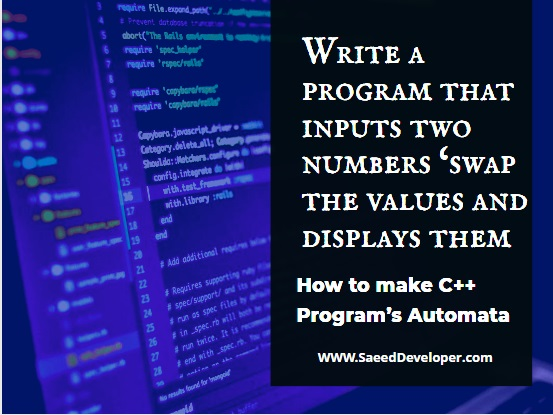# Q1 Write a program that inputs two numbers ‘swap the values and displays them.

## Also, Make the Automata of C++ ProgramWrite a program that inputs two numbers ‘swap values and displays

Hi, there in this article I will write a program that will input the  2 numbers ‘ and then I will swap the values and then displays the output of the program. So let's see the solution. Write a program that inputs two numbers ‘swap values and displays all values.

### Here is the answer of the question, write a program that inputs two numbers ‘swap the values and displays them also making the automata of C++ program

#include<iostream.h>
#include<conio.h>
// Global variables
Int x;
Int y;
Int z;
Void take data ();
void process data ();
Void show data ();
Void main ()
{
Take data ();
Process data ();
Show data ();
}
Void take data ()
{
Cout <<” input taken from user “;
}
Void process data ()
{
Cout <<” swapping the values of the numbers “;
}
Void show data ()
{

Cout << “display the swap numbers “ ;
}

### Here are the automata of the C++ program2
a                       b                           c
 1
 3
 4

x     1’2 =   take data from the user.
y     2,3 = process on the data
z     3’4 = show data / result
1 is the initial state
4 is the ending state

## How does this program work?

In this short and C++ programming tutorial, I tried to show you how to work with C++ using Automata. In this simple program, I just want to show you how to work with variables and many more things.
The theory of automata shows how automatically works the programs and C++ programs show how to work accordingly.
In this program, the variables will show their data with the Cout function to displays the data. void take data function makes enable the user to put data into the program. Void process data function processing the data.
Void Show data function shows data in the program.